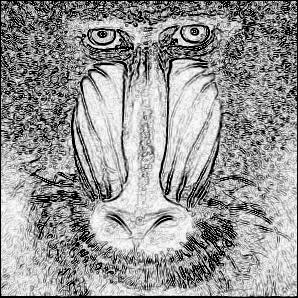# 3.1   Using Data Types

A data type is a set of values and a set of operations defined on those values. The primitive data types that you have been using are supplemented in Java by extensive libraries of reference types that are tailored for a large variety of applications. In this section, we consider reference types for string processing and image processing.

## Strings.

You have already been using a data type that is not primitive—the String data type, whose values are sequences of characters. We specify the behavior of a data type in an application programming interface (API). Here is a partial API for Java’s String data type: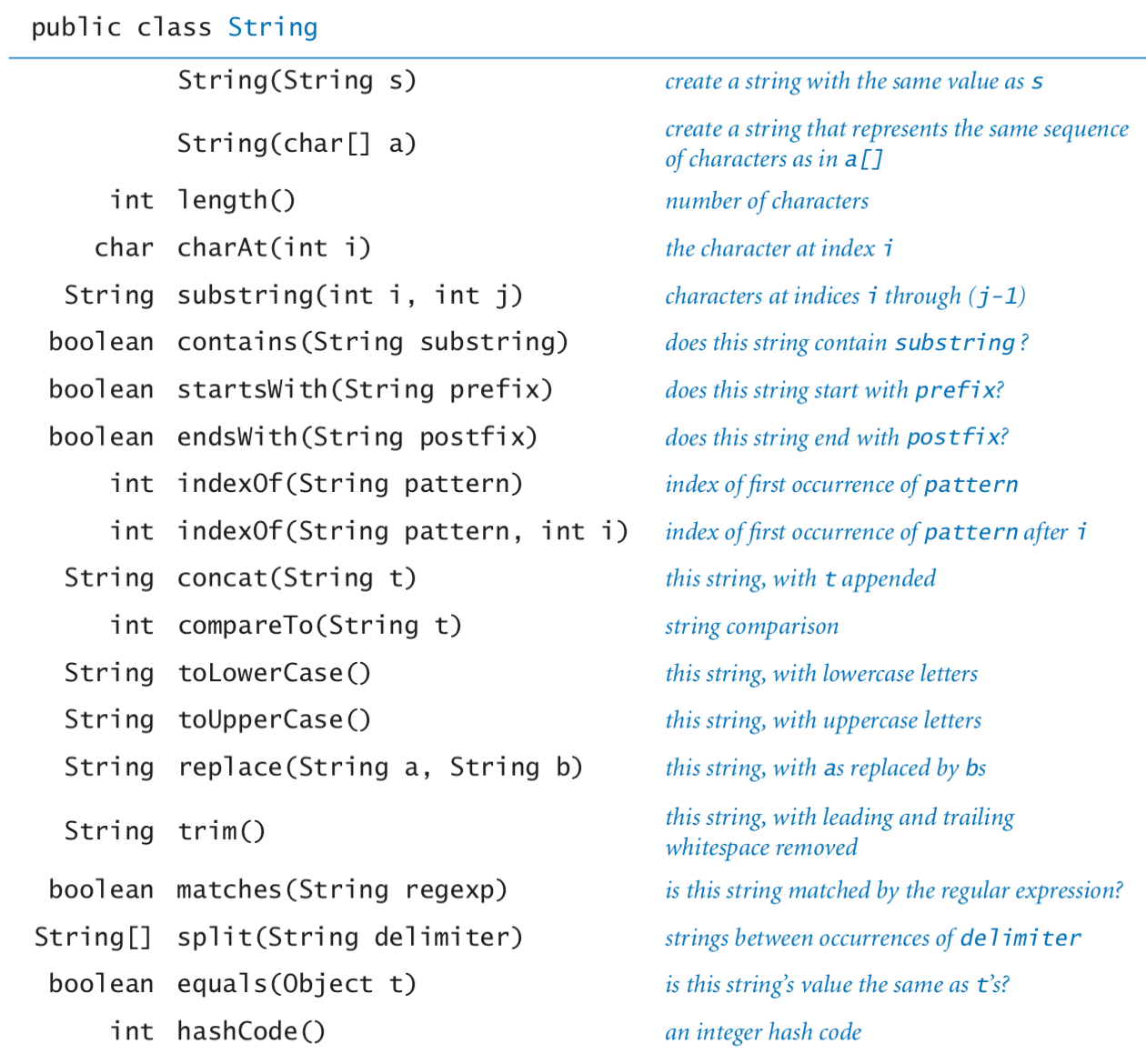The first entry, with the same name as the class and no return type, defines a special method known as a constructor. The other entries define instance methods that can take arguments and return values.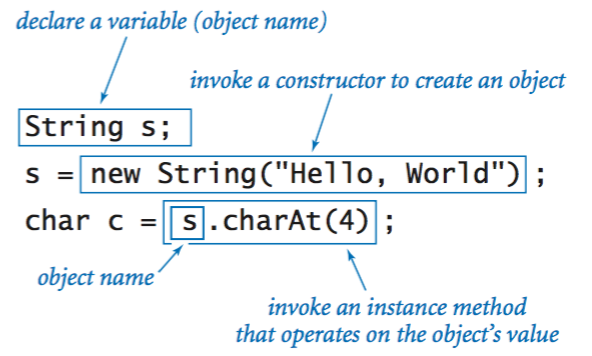• Declaring variables. You declare variables of a reference type in precisely the same way that you declare variables of a primitive type. A declaration statement does not create anything; it just says that we will use the variable name s to refer to a String object.
• Creating objects. Each data-type value is stored in an object. When a client invokes a constructor, the Java system creates (or instantiates) an individual object (or instance). To invoke a constructor, use the keyword new; followed by the class name; followed by the constructor’s arguments, enclosed in parentheses and separated by commas.
• Invoking instance methods. The most important difference between a variable of a reference type and a variable of a primitive type is that you can use reference-type variables to invoke the instance methods that implement data-type operations (in contrast to the built-in syntax involving operators such as +* that we used with primitive types).
Now, we consider various string-processing examples.
• Data-type operations. The following examples illustrate various operations for the String data type.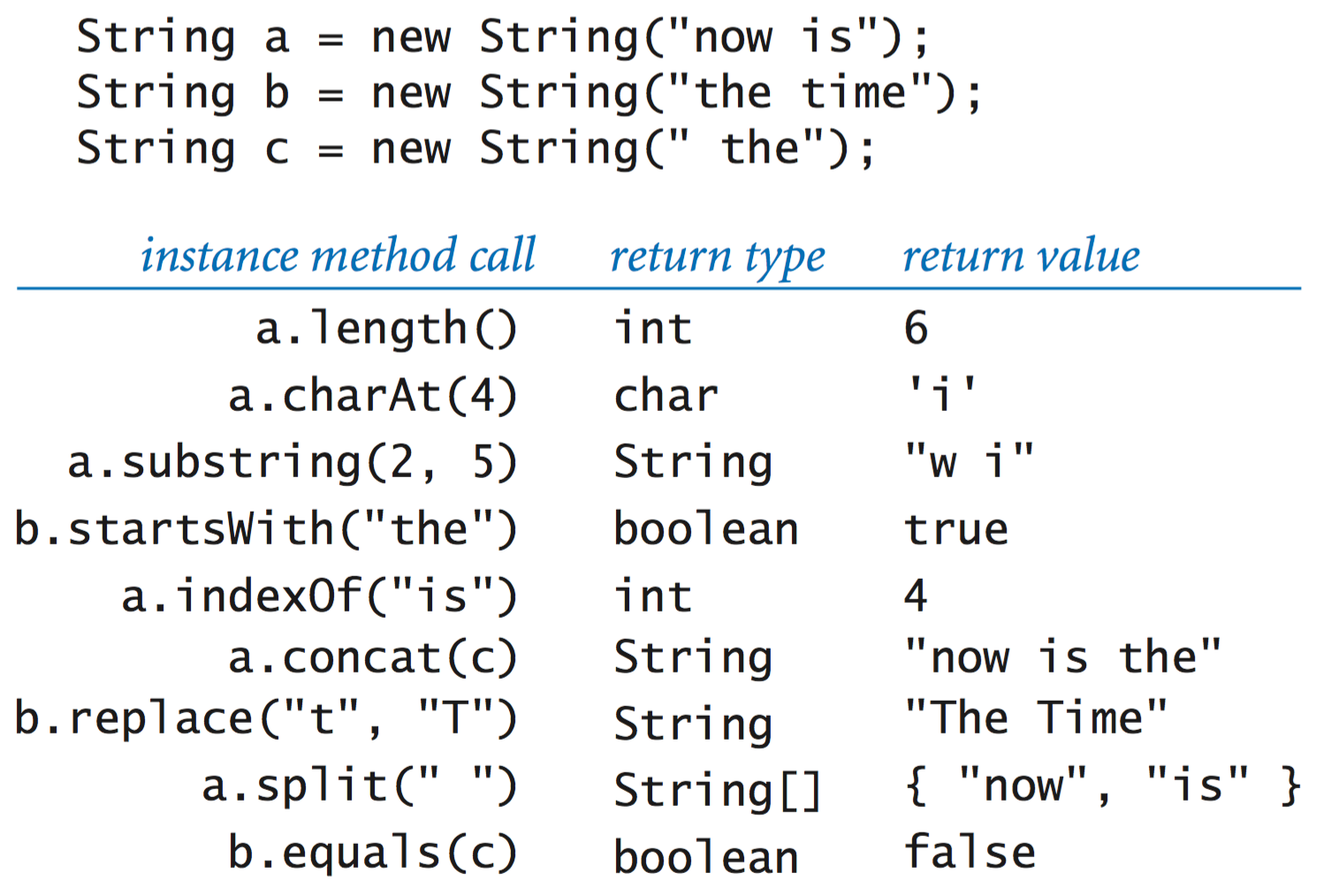• Code fragments. The following code fragments illustrate the use of various string-processing methods.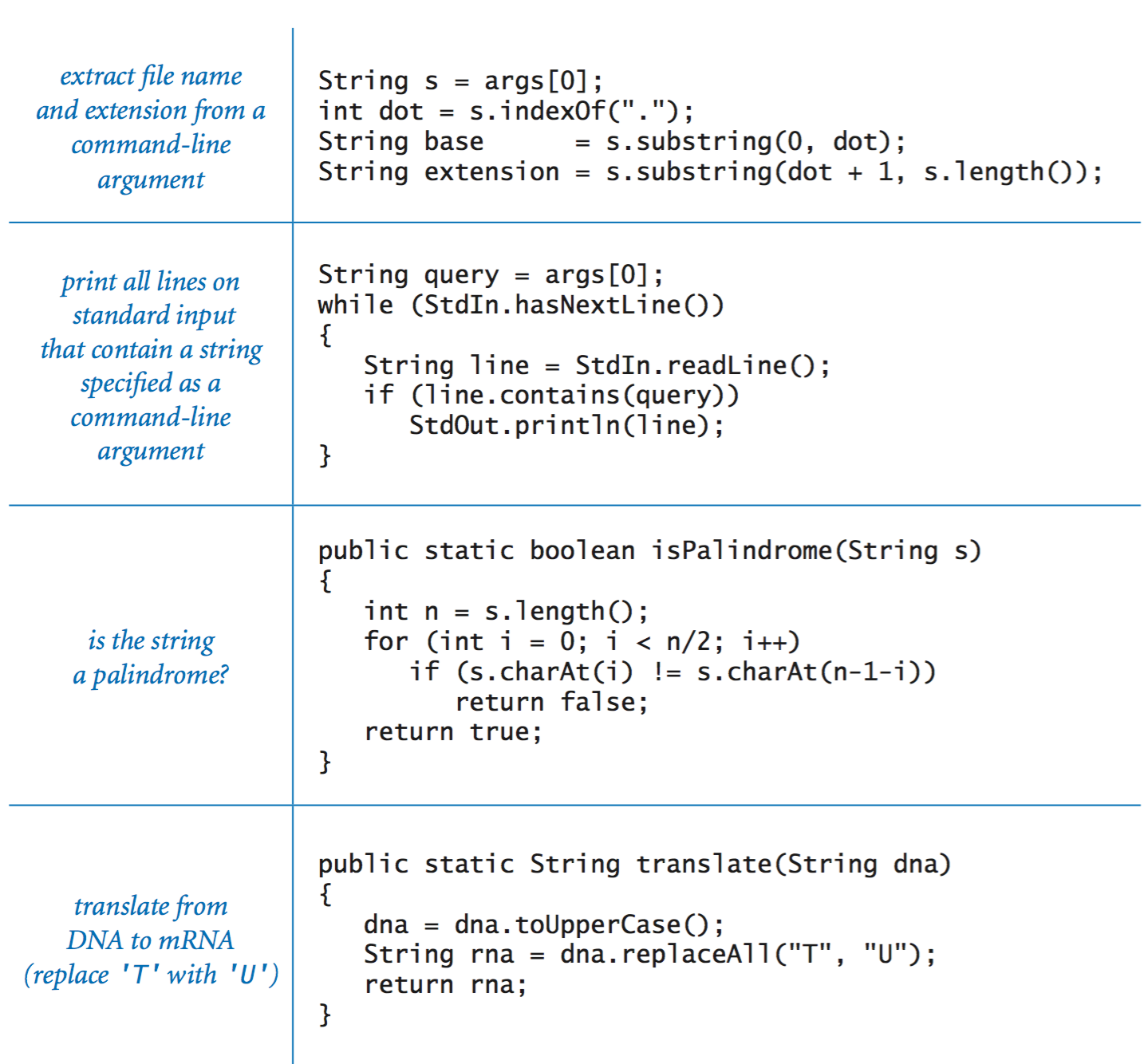• Genomics. Biologists use a simple model to represent the building blocks of life, in which the letters A, C, G, and T represent the four bases in the DNA of living organisms. A gene is a substring that represents a functional unit of critical importance in understanding life processes. PotentialGene.java takes a DNA string as an argument and determines whether it corresponds to a potential gene based on the following criteria:
• It begins with the start codon ATG.
• Its length is a multiple of 3.
• It ends with one of the stop codons TAG, TAA, or TGA.
• It has no intervening stop codons.

## Color.Java's Color data type represents color values using the RGB color model where a color is defined by three integers (each between 0 and 255) that represent the intensity of the red, green, and blue components of the color. Other color values are obtained by mixing the red, blue and green components.

The Color data type has a constructor that takes three integer arguments. For example, you can write

 Color red = new Color(255, 0, 0); Color bookBlue = new Color( 9, 90, 166); 
to create objects whose values represent pure red and the blue used to print this book. The following table summarizes the methods in the Color API that we use in this book: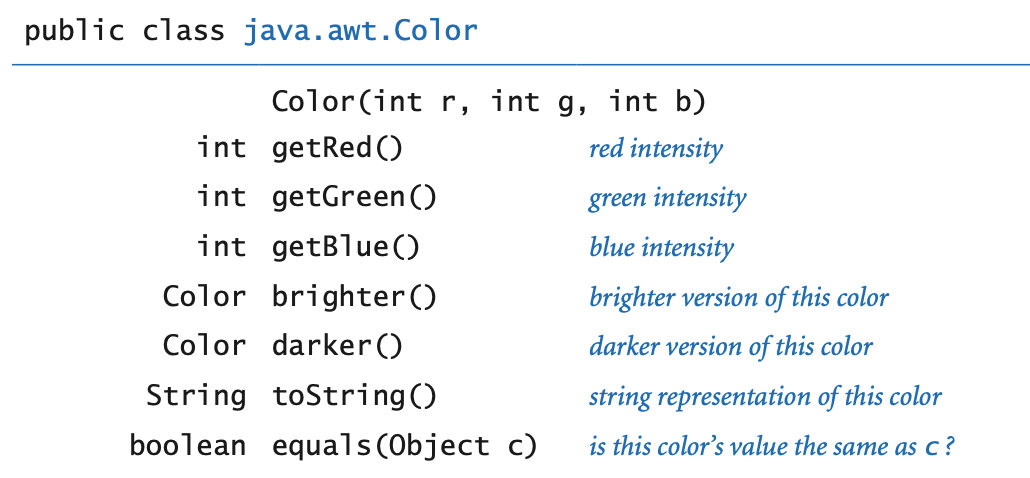Here are some example clients that use the Color data type.
• Albers squares. AlbersSquares.java displays the two colors entered in RGB representation on the command line in the format developed in the 1960s by Josef Albers that revolutionized the way that people think about color.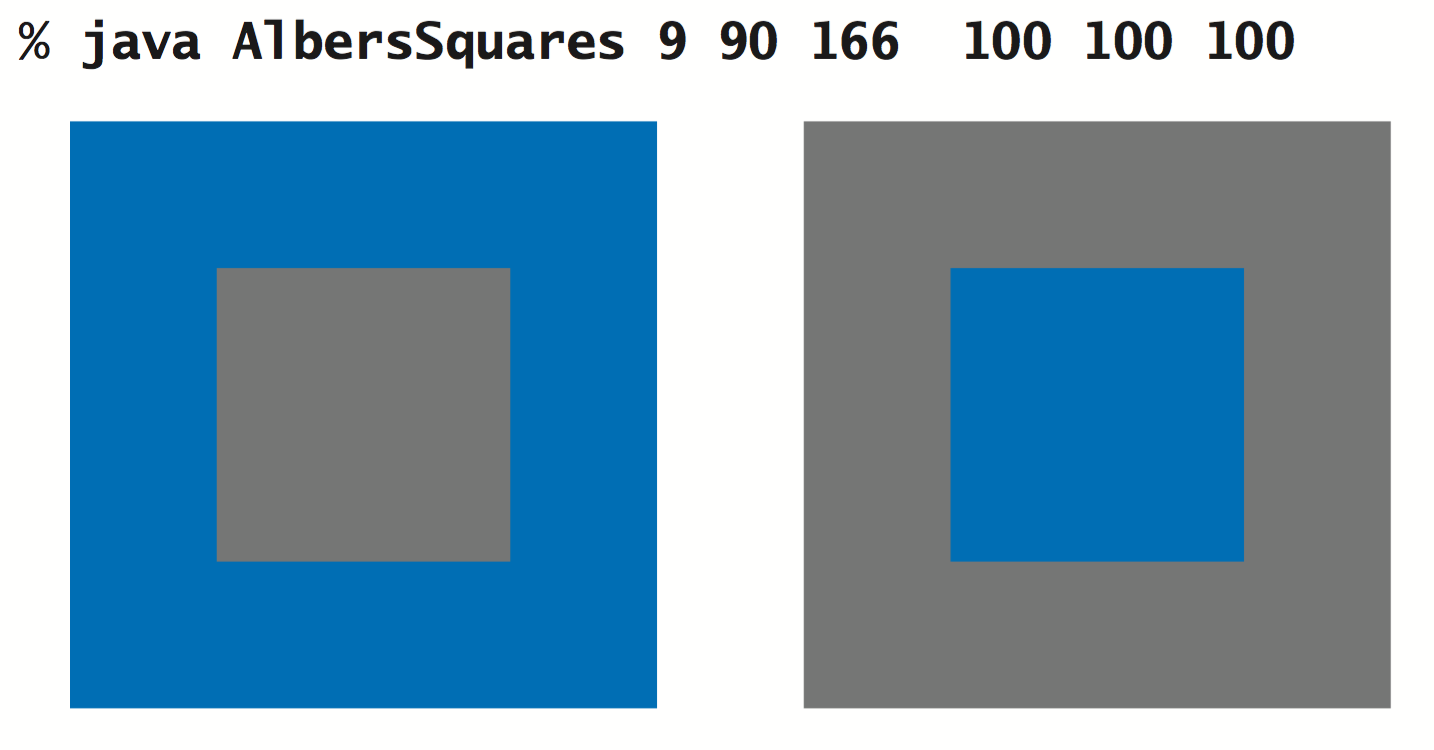• Luminance. The quality of the images on modern displays such as LCD monitors, plasma TVs, and cell-phone screens depends on an understanding of a color property known as monochrome luminance, or effective brightness. It is a linear combination of the three intensities: if a color's red, green and blue values are r, g, and b, respectively then its luminance is defined by the equation
$$Y = 0.299r + 0.587g + 0.114b$$
• Grayscale.The RGB color model has the property that when all three color intensities are the same, the resulting color is on a grayscale that ranges from black (all 0s) to white (all 255s). A simple way to convert a color to grayscale is to replace the color with a new one whose red, green, and blue values equal its luminance.
• Color compatibility. The luminance value is also crucial in determining whether two colors are compatible, in the sense that printing text in one of the colors on a background in the other color will be readable. A widely used rule of thumb is that the difference between the luminance of the foreground and background colors should be at least 128.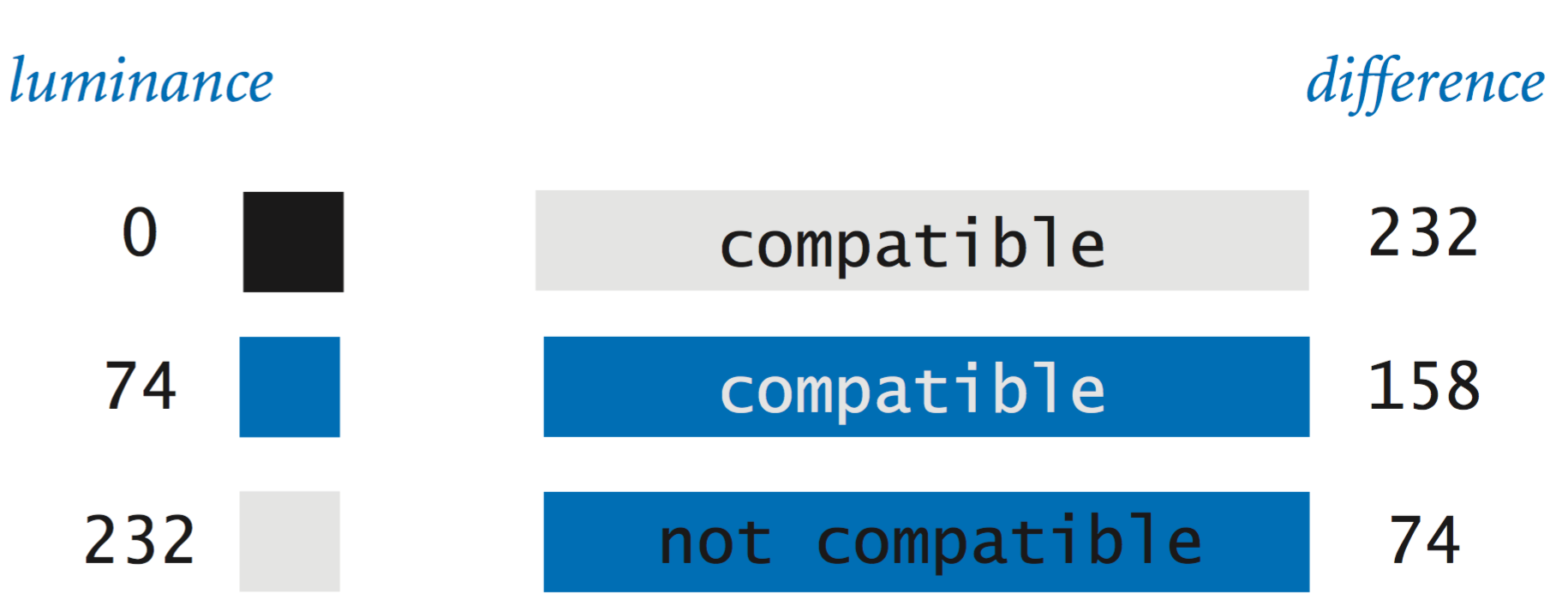Luminance.java is a static method library that we can use to convert to grayscale and test whether two colors are compatible.

## Image processing.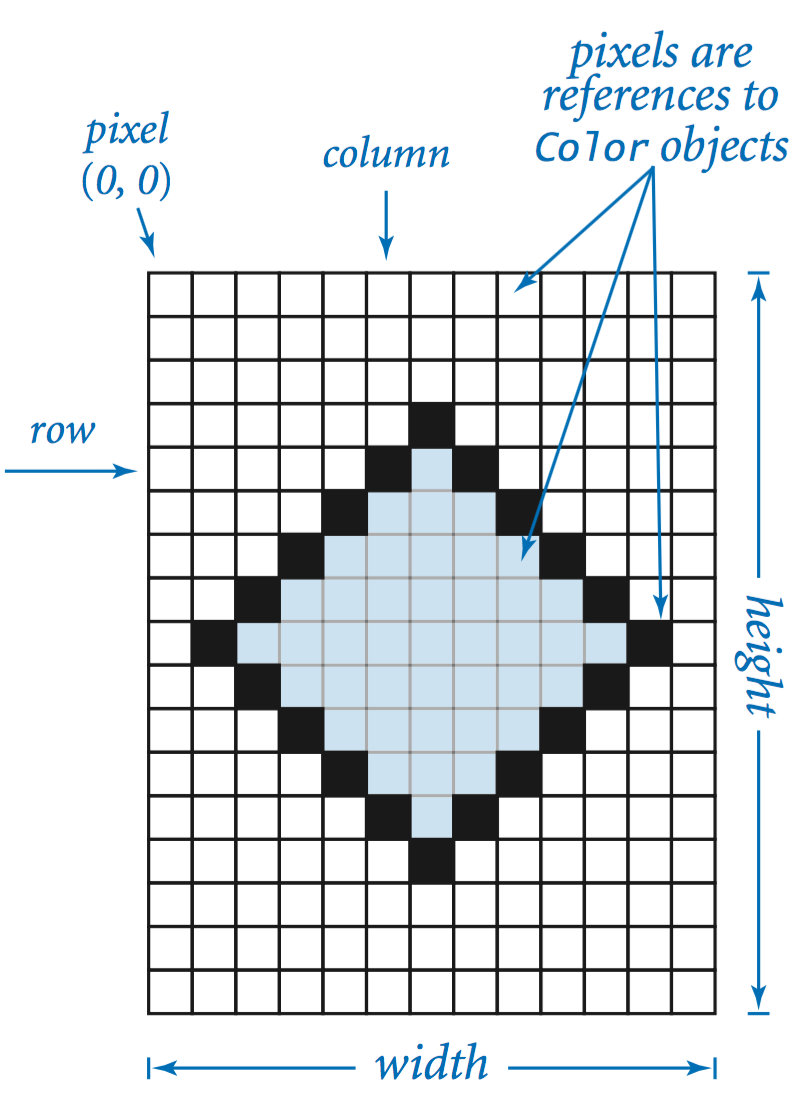A digital image is a rectangular grid of pixels (picture elements), where the color of each pixel is individually defined. Digital images are sometimes referred to as raster or bitmapped images. In contrast, the types of images that we produce with StdDraw (which involved geometric objects) are referred to as vector images.

The Picture data type allows you to manipulate digital images. The set of values is a two-dimensional matrix of Color values, and the operations are what you might expect: create an image (either blank or from a file), set the value of a pixel to a given color, and extract the color of a given pixel. The following API summarizes the available operations: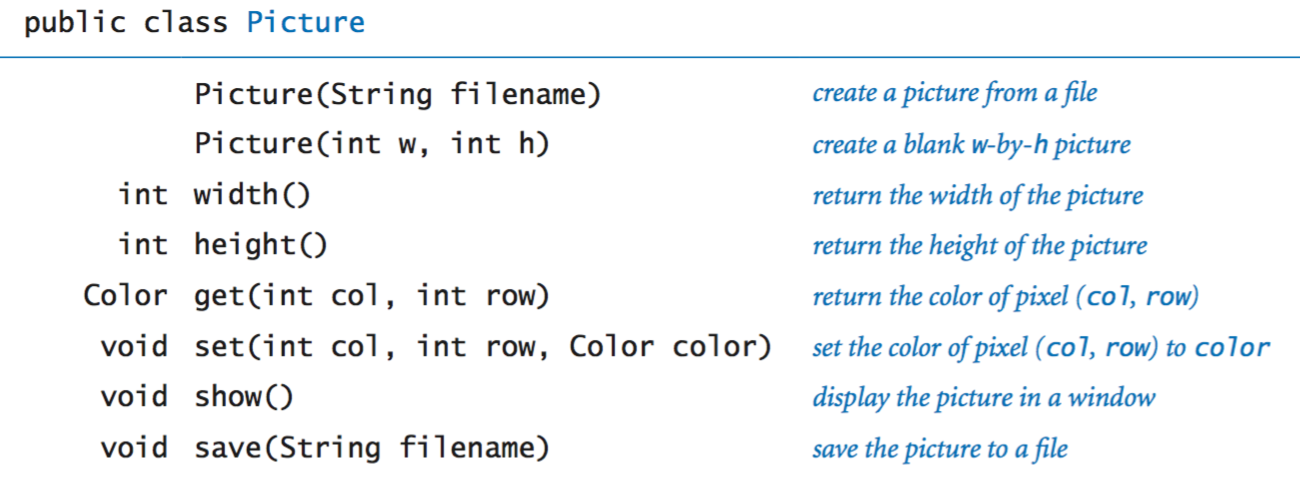Most image-processing programs are filters that scan through all of the pixels in a source image and then perform some computation to determine the color of each pixel in a target image.
• Grayscale. Grayscale.java converts an image from color to grayscale.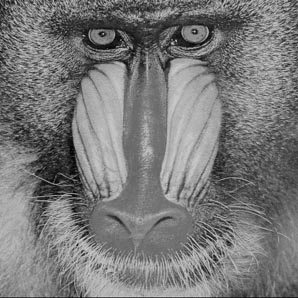• Scale. Scale.java takes the name of an image file and two integers (width w and height h) as command-line arguments, scales the picture to w-by-h, and displays both images.600-by-300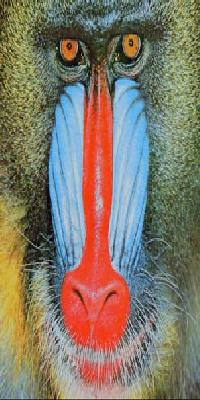200-by-400

• Fade effect. Fade.java takes an integer n and the names of the source and target images as command-line arguments and fades from the source image to the target image in n steps. It uses a linear interpolation strategy, where each pixel in image i is a weighted average of the corresponding pixels in the source and target images.

## Input and output revisited.

In Section 1.5 you learned how to read and write numbers and text using standard input, output, and drawing. These restrict us to working with just one input file, one output file, and one drawing for any given program. With object-oriented programming, we consider data types that allow us to work with multiple input streams, output streams, and drawings within one program.
• Input stream data type. In is an object-oriented version of StdIn that supports reading numbers and text from data from files and websites as well as the standard input stream.• Output stream data type. Out is an object-oriented version of StdOut that supports printing text to a variety of output streams, including files and standard output.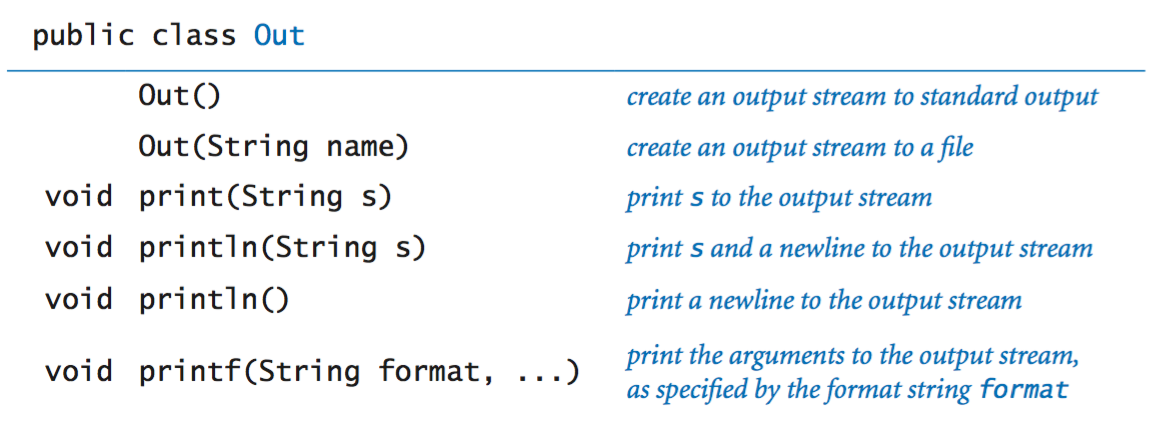• File concatenation. Cat.java reads several files specified as command-line arguments, concatenates them, and prints the result to a file.
• Screen scraping. StockQuote.java takes the the symbol of New York Stock Exchange stock as a command-line argument and prints its current trading price. It uses a technique known as screen scraping, in which the goal is to extract some information from a web page with a program. To report the current stock price of Google (NYSE symbol = GOOG), it reads the Web page http://finance.yahoo.com/quote/GOOG. Then, it identifies the relevant information using indexOf() and substring().
• Extracting data. Split.java uses multiple output streams to split a CSV file into separate files, one for each comma-delimited field.
• Drawing data type. Draw is an object-oriented version of StdDraw that supporting drawing to more than one canvas in the same program.

## Properties of reference types.

We summarize some of the essential properties of reference types.
• Aliasing. An assignment statement with a reference type creates a second copy of the reference. The assignment statement does not create a new object, just another reference to an existing object. This situation is known as aliasing: both variables refer to the same object. As an example, consider the following code fragment:
 Picture a = new Picture("mandrill.jpg"); Picture b = a; a.set(col, row, color1); // a updated b.set(col, row, color2); // a updated again 

After the second assignment statement, variables a and b refer to the same Picture object.

• Pass by value. When you call a method with arguments, the effect in Java is as if each argument were to appear on the right-hand side of an assignment statement with the corresponding argument name on the left-hand side. That is, Java passes a copy of the argument value from the caller to the method. If the argument value is a primitive type, Java passes a copy of that value; if the argument value is an object reference, Java passes a copy of the object reference. This arrangement is known as pass by value.
• Arrays are objects. In Java, arrays are objects. As with strings, special language support is provided for certain operations on arrays: declarations, initialization, and indexing. As with any other object, when we pass an array to a method or use an array variable on the right-hand side of an assignment statement, we are making a copy of the array reference, not a copy of the array.
• Arrays of objects. When we create an array of objects, we do so in two steps:
• Create the array by using new and the square bracket syntax for array creation.
• Create each object in the array, by using new to call a constructor.

For example, the following code creates an array of two Color objects:

 Color[] a = new Color; a = new Color(255, 255, 0); a = new Color(160, 82, 45); 
• Safe pointers. In Java, there is only one way to create a reference (with new) and only one way to manipulate that reference (with an assignment statement). Java references are known as safe pointers, because Java can guarantee that each reference points to an object of the specified type (and not to an arbitrary memory address).
• Orphaned objects. The ability to assign different objects to a reference variable creates the possibility that a program may have created an object that it can no longer reference. Such an object is said to be orphaned. As an example, consider the following code fragment:
 Color a, b; a = new Color(160, 82, 45); // sienna b = new Color(255, 255, 0); // yellow b = a; 
After the final assignment statement, not only do a and b refer to the same Color object (sienna), but also there is no longer a reference to the Color object that was created and usedto initialize b (yellow).
• Garbage collection. One of Java’s most significant features is its ability to automatically manage memory. The idea is to free the programmer from the responsibility of managing memory by keeping track of orphaned objects and returning the memory they use to a pool of free memory. Reclaiming memory in this way is known as garbage collection, and Java’s safe pointer policy enables it to do this efficiently.

#### Exercises

1. Write a function reverse() that takes a string as an argument and returns a string that contains the same sequence of characters as the argument string but in reverse order.

Solution: ReverseString.java.

2. Write a program FlipX.java that takes the name of an image file as a command-line argument and flips the image horizontally.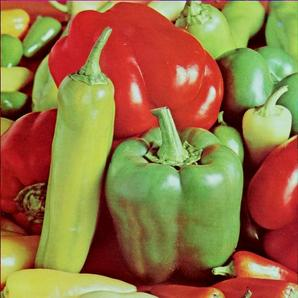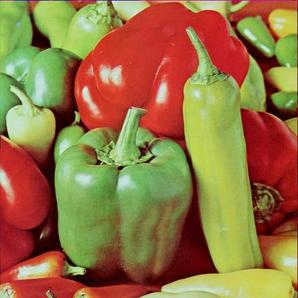3. Write a program ColorSeparation.java that takes the name of an image file as a command-line argument, and creates and shows three Picture objects, one that contains only the red components, one for green, and one for blue.4. Write a static method isValidDNA() that takes a string as its argument and returns true if and only if it is composed entirely of the characters A, T, C, and G.

Solution:

 public static boolean isValidDNA(String s) { for (int i = 0; i < s.length(); i++) { char c = s.charAt(i); if (c != 'A' && c != 'T' && c != 'C' && c != 'G') return false; } 
5. Write a function complementWatsonCrick() that takes a DNA string as its arguments and returns its Watson–Crick complement: replace A with T, C with G, and vice versa.

Solution:

 public static String complementWatsonCrick(String s) { s = s.replaceAll("A", "t"); s = s.replaceAll("T", "a"); s = s.replaceAll("C", "g"); s = s.replaceAll("G", "c"); return s.toUpperCase(); } 
6. What does the following code fragment print?
 String string1 = "hello"; String string2 = string1; string1 = "world"; System.out.println(string2); 

Solution: hello.

7. What does the following code fragment print?
 String s = "Hello World"; s.toUpperCase(); s.substring(6, 11); StdOut.println(s); 

Solution: Hello, World. String objects are immutable.

8. A string s is a circular shift of a string t if it matches when the characters of one string are circularly shifted by some number of positions. For example, ACTGACG is a circular shift of TGACGAC, and vice versa. Detecting this condition is important in the study of genomic sequences. Write a function isCircularShift() that checks whether two given strings s and t are circular shifts of one another.

Solution:

 public boolean isCircularShift(String s, String t) { String s2 = s + s; return s2.contains(t); } 
9. What does the following recursive function return?
 public static String mystery(String s) { int n = s.length(); if (n <= 1) return s; String a = s.substring(0, n/2); String b = s.substring(n/2, N); return mystery(b) + mystery(a); } 

Solution: the reverse of its argument string.

10. Suppose that a[] and b[] are both integer arrays consisting of millions of integers. What does the follow code do, and how long does it take?
 int[] temp = a; a = b; b = temp; 

Solution: It swaps the arrays, but it does so by copying object references, so that it is not necessary to copy millions of values.

11. Describe the effect of the following function.
 public void swap(Color a, Color b) { Color temp = a; a = b; b = temp; } 

Solution: It has no effect because Java passes object references by value.

#### Creative Exercises

1. Kamasutra cipher. Write a filter KamasutraCipher.java that takes two strings as command-line argument (the key strings), then reads strings (separated by whitespace) from standard input, substitutes for each letter as specified by the key strings, and prints the result to standard output. This operation is the basis for one of the earliest known cryptographic systems. The condition on the key strings is that they must be of equal length and that any letter in standard input must appear in exactly one of them. For example, if the two keys are THEQUICKBROWN and FXJMPSVLAZYDG, then we make the table
 T H E Q U I C K B R O W N F X J M P S V L A Z Y D G 
which tells us that we should substitute F for T, T for F, H for X, X for H, and so forth when filtering standard input to standard output. The message is encoded by replacing each letter with its pair. For example, the message MEET AT ELEVEN is encoded as QJJF BF JKJCJG. The person receiving the message can use the same keys to get the message back.
2. Color study. Write a program ColorStudy.java that displays the color study shown at right, which gives Albers squares corresponding to each of the 256 levels of blue (blue-to-white in row-major order) and gray (black-to-white in column-major order) that were used to print this book.3. Tile. Write a program Tile.java that takes the name of an image file and two integers m and n as command-line arguments and creates an m-by-n tiling of the image.4. Rotation filter. Write a program Rotation.java that takes two command-line arguments (the name of an image file and a real number $$\theta$$) and rotates the image $$\theta$$) degrees counterclockwise. To rotate, copy the color of each pixel $$(s_i, s_j)$$ in the source image to a target pixel $$(t_i, t_j)$$ whose coordinates are given by the following formulas:
\begin{align} t_i \;&=\; (s_i - c_j) \cos \theta - (s_j - c_j) \sin \theta + c_j \\[1ex] t_j \;&=\; (s_i - c_j) \sin \theta + (s_j - c_j) \cos \theta + c_j \end{align}
where $$(c_i, c_j)$$ is the center of the image.5. Swirl filter. Creating a swirl effect is similar to rotation, except that the angle changes as a function of distance to the center of the image. Use the same formulas as in the previous exercise, but compute $$\theta$$ as a function of $$(s_i, s_j)$$, specifically $$\pi/256$$ times the distance to the center.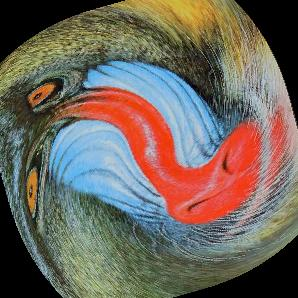6. Wave filter. Write a filter Wave.java like those in the previous two exercises that creates a wave effect, by copying the color of each pixel $$(s_i, s_j)$$ in the source image to a target pixel $$(t_i, t_j)$$, where
\begin{align} t_i \;&=\; s_i \\[1ex] t_j \;&=\; s_j + 20 \sin(2 \pi s_j / 64) \end{align}
Add code to take the amplitude (20 in the accompanying figure) and the frequency (64 in the accompanying figure) as command-line arguments.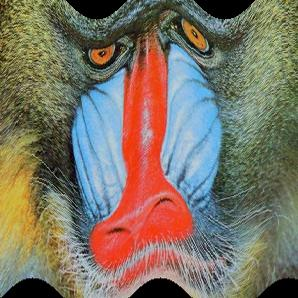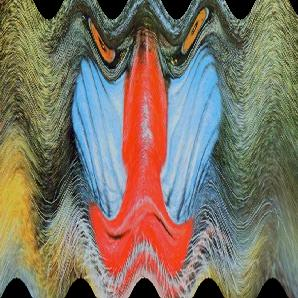7. Glass filter. Write a program Glass.java that takes the name of an image file as a command-line argument and applies a glass filter: set each pixel p to the color of a random neighboring pixel (whose pixel coordinates both differ from p’s coordinates by at most 5).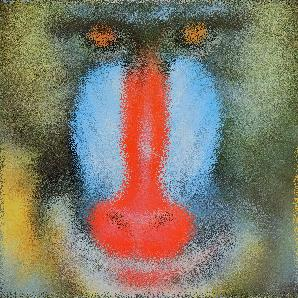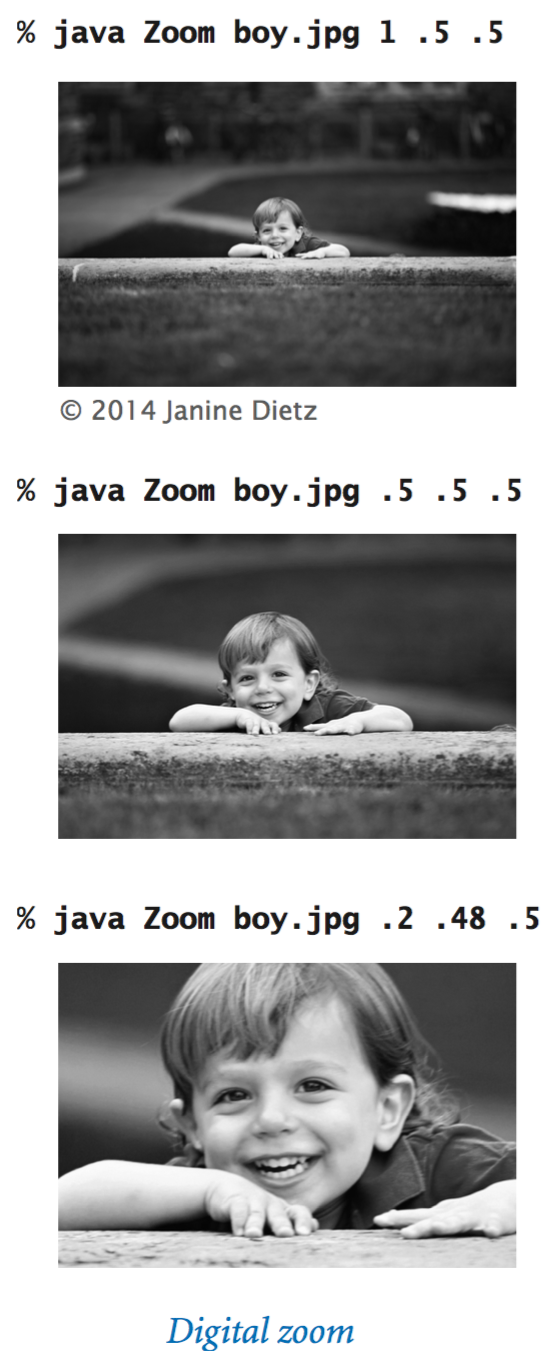8. Digital zoom. Write a program Zoom.java that takes the name of an image file and three numbers s, x, and y as command-line arguments, and shows an output image that zooms in on a portion of the input image. The numbers are all between 0 and 1, with s to be interpreted as a scale factor and (x, y) as the relative coordinates of the point that is to be at the center of the output image. Use this program to zoom in on a relative or pet in some digital photo on your computer.

#### Web Exercises (String Processing)

1. Write a function that takes as input a string and returns the number of occurrences of the letter e.
2. Give a one line Java code fragment to replace all periods in a string with commas. Answer: s = s.replace('.', ',').

Don't use s = s.replaceAll(".", ","). The replaceAll() method uses regular expressions where "."has a special meaning.

3. Replace all tabs with four spaces. Answer: s = s.replace("\t", "    ").
4. Write a program that takes a command line input string s, reads strings from standard input, and prints the number of times s appears. Hint: use don't forget to use equals instead of == with references.
5. Write a program that reads in the name of a month (3 letter abbreviation) as a command-line argument and prints the number of days in that month in a non leap year.
  public static void main(String[] args) { String[] months = { "Jan", "Feb", "Mar", "Apr", "May", "Jun", "Jul", "Aug", "Sep", "Oct", "Nov", "Dec" }; int[] days = { 31, 28, 31, 30, 31, 30, 31, 31, 30, 31, 30, 31 }; String name = args; for (int i = 0; i < months.length; i++) if (name.equalsIgnoreCase(months[i])) System.out.println(name + " has " + days[i] + " days"); } 

6. Write a program Squeeze.java that takes as input a string and removes adjacent spaces, leaving at most one space in-a-row.
7. Which one or more of the following converts all of the strings in the array a to upper case?
 for (int i = 0; i < a.length; i++) { String s = a[i]; s = s.toUpperCase(); } for (int i = 0; i < a.length; i++) { a[i].toUpperCase(); } for (int i = 0; i < a.length; i++) { a[i] = a[i].toUpperCase(); } 
8. Describe the string that the following function returns, given a positive integer n?
 public static String mystery(int n) { String s = ""; while (n > 0) { if (n % 2 == 1) s = s + s + "x"; else s = s + s; n = n / 2; } return s; } 

Solution The string of length n consisting only of the character x.

9. Write a function that takes a string s and an integer n and returns a new string t of length exactly n that consists of s (truncated if its length is greater than n) followed by a sequence of '-' characters (if the length of s is less than n).
10. What does the following recursive function return, given two strings s and t of the same length?
 public static String mystery(String s, String t) { int n = s.length(); if (n <= 1) return s + t; String a = mystery(s.substring(0, n/2), t.substring(0, n/2)); String b = mystery(s.substring(n/2, n), t.substring(n/2, n)); return a + b; } 

Solution: Perfect shuffle of the characters of s and t.

11. Write a program that reads in a string and prints the first character that appears exactly once in the string. Ex: ABCDBADDAB -> C.
12. Given a string, create a new string with all the consecutive duplicates removed. Ex: ABBCCCCCBBAB -> ABCBAB.
13. Write a function that takes two string arguments s and t, and returns the index of the first character in s that appears in ts (or -1 if no character in s appears in t).
14. Given a string s, determine whether it represents the name of a web page. Assume that any string starting with http:// is a web page.

Solution: if (s.startsWith("http://")).

15. Given a string s that represents the name of a web page, break it up into pieces, where each piece is separated by a period, e.g., http://www.cs.princeton.edu should be broken up into www, cs, princeton, and edu, with the http:// part removed. Use either the split() or indexOf() methods.
16. Given a string s that represents the name of a file, write a code fragment to determine its file extension. The file extension is the substring following the last period. For example, the file type of monalisa.jpg is jpg, and the file type of mona.lisa.png is png.

Library solution: this solution is used in Picture.java to save an image to the file of the appropriate type.

 String extension = s.substring(s.lastIndexOf('.') + 1); 

17. Given a string s that represents the name of a file, write a code fragment to determine its directory portion. This is the prefix that ends with the last / character (the directory delimiter); if there is no such /, then it is the empty string. For example, the directory portion of /Users/wayne/monalisa.jpg is /Users/wayne/.
18. Given a string s that represents the name of a file, write a code fragment to determine its base name (filename minus any directories). For /Users/wayne/monalisa.jpg, it is monalisa.jpg.
19. Write a program that reads in text from standard input and prints it back out, replacing all single quotation marks with double quotation marks.
20. Write a program Paste.java that takes an arbitrary number of command line inputs and concatenates the corresponding lines of each file, and writes the results to standard output. (Typically each line in given file has the same length.) Counterpart of the program Cat.java.
21. What does the program LatinSquare.java print when N = 5?
 String alphabet = "ABCDEFGHIJKLMNOPQRSTUVWXYZ"; for (int i = 0; i < N; i++) { for (int j = 0; j < N; j++) { char c = alphabet.charAt((i + j) % N); System.out.print(c + " "); } System.out.println(); } 

A Latin square of order N is an N-by-N array consisting of N different symbols, such that each symbol appears exactly once in each row and column. Latin squares are useful in statistical design and cryptography.

22. What does the following code fragment print?
 String s = "Hello World"; s.toUpperCase(); s.substring(6, 11); System.out.println(s); 

Answer: Hello World. The methods toUpperCase and substring return the resulting strings, but the program ignores these so s is never changed. To get it to print WORLD, use s = s.toUpperCase() and s = s.substring(6, 11).

23. What happens when you execute the following code fragment?
 String s = null; int length = s.length(); 

Answer: you get a NullPointerException since s is null and you are attempting to dereference it.

24. What are the values of x and y after the two assignment statements below?
 int x = '-'-'-'; int y = '/'/'/'; 
25. What does the following statement do where c if of type char?
 System.out.println((c >= 'a' && c <= 'z') || (c >= 'A' && c <= 'Z')); 
Answer: prints true if c is an uppercase or lowercase letter, and false otherwise.
26. Write an expression that tests whether or not a character represents one of the digits '0' through '9' without using any library functions.
 boolean isDigit = ('0' <= c && c <= '9'); 

27. Write a program WidthChecker.java that takes a command line parameter N, reads text from standard input, and prints to standard output all lines that are longer than N characters (including spaces).
28. Write a program Hex2Decimal.java that converts from a hexadecimal string (using A-F for the digits 11-15) to decimal.
29. wget. Write a program Wget.java that takes the name of a URL as a command-line argument and saves the referenced file using the same filename.
30. Capitalize. Write a program Capitalize.java that reads in text from standard input and capitalizes each word (make first letter uppercase and make the remaining letters lowercase).
31. Shannon's entropy experiment. Recreate Shannon's experiment on the entropy of the English language by listing a number of letters in a sentence and prompting the user for the next symbol. Shannon concluded that there is approximately 1.1 bits of info per letter in the alphabet.
32. Scrambled text. Some cognitive psychologists believe that people recognize words based on their shape.
to a rscheearch at an Elingsh uinervtisy, it deosn't mttaer in waht oredr the ltteers in a wrod are, the olny iprmoetnt tihng is taht frist and lsat ltteer is at the rghit pclae. The rset can be a toatl mses and you can sitll raed it wouthit porbelm. Tihs is bcuseae we do not raed ervey lteter by itslef but the wrod as a wlohe.
Write a program that reads in text from standard input and prints the text back out, but shuffles the internal letters in each word. Write and use a function scramble() that takes as input a string and returns another string with the internal letters in random order. Use the shuffling algorithm in Shuffle.java for the shuffling part.
33. Date format conversion. Write a program to read in a data of the form 2003-05-25 and convert it to 5/25/03.
34. Frequency analysis of English text. Write a program LetterFrequency.java that reads in text from standard input (e.g., Moby Dick) and calculate the fraction of times each of the 26 lowercase letters appears. Ignore uppercase letters, punctuation, whitespace, etc. in your analysis. Use CharStdIn.java from Section 2.4 to read process the text file.
35. Print longest word(s). Repeat the previous exercise, but print out all of the longest words if there is a tie, say up to a maximum of 10 words. Use an array of strings to store the current longest words.
36. Test if two files are equal. Write a program that takes the name of two text files as command line inputs and checks if their contents are identical.
37. Parsing command-line options. Unix command line programs typically support flags which configure the behavior of a program to produce different output, e.g., "wc -c". Write a program that takes any number of flags from the command line and runs whichever options the user specifies. To check options, use something like if (s.equals("-v")).
38. Capitalization. Write a program Capitalizer.java that reads in text strings from standard input and modifies each one so that the first letter in each word is uppercase and all other letters are lowercase.
39. Railfence transposition cipher. Write a program RailFenceEncoder.java that reads in text from standard input and prints the characters in the odd positions, followed by the even positions. For example, if the original message is "Attack at Dawn", then you should print out "Atc tDwtaka an". This is a crude form of cryptography.
40. Railfence transposition cipher. Write a program RailFenceDecoder.java that reads in a message encoded using the railfence transposition cipher and prints the original message by reversing the encryption process.
41. Scytale cipher. The scytale cipher is one of the first cryptographic devices used for military purposes. (See The Code Book, p. 8 for a nice picture.) It was used by the Spartans in the fifth century BCE. To scramble the text, you print out every kth character starting at the beginning, then every kth character starting at the second character, and so forth. Write a pair of programs ScytaleEncoder.java and ScytaleDecoder.java that implement this encryption scheme.
42. Print longest word. Read a list of words from standard input, and print out the longest word. Use the length method.
43. Subsequence. Given two strings s and t, write a program Subsequence.java that determines whether s is a subsequence of t. That is, the letters of s should appear in the same order in t, but not necessarily contiguously. For example accag is a subsequence of taagcccaaccgg.
44. Bible codes. Some religious zealots believe that the Torah contains hidden phrases that appear by reading every kth letter, and that such pattern can be used to find the Ark of the Covenant, cure cancer, and predict the future. Results not based on scientific method and results have been debunked by mathematicians and attributed to illicit data manipulation. Using the same methodology one can find statistically similar patterns in a Hebrew translation of War and Peace.
45. Word chain checker. Write a program that reads in a list of words from the command line and prints true if they form a word chain and false otherwise. In a word chain, adjacent words must differ in exactly one letter, e.g., HEAL, HEAD, DEAD, DEED, DEER, BEER.
46. Haiku detector. Write a program that reads in text from standard input and checks whether it forms a haiku. A haiku consists of three lines containing the correct number of syllables (5, 7, and 5, respectively). For the purpose of this problem, define a syllable to be any contiguous sequence of consecutive vowels (a, e, i, o, u, or y). According to this rule, haiku has two syllables and purpose has three syllables. Of course, the second example is wrong since the e in purpose is silent.
47. ISBN numbers. Write a program to check whether an ISBN number is valid. Recall check digit. An ISBN number can also have hyphens inserted at arbitrary places.
48. Longest common prefix. Write a function that takes two input string s and t, and returns the longest common prefix of both strings. For example, if s = ACCTGAACTCCCCCC and t = ACCTAGGACCCCCC, then the longest common prefix is ACCT. Be careful if s and t start with different letters, or if one is a prefix of the other.
49. Longest complemented palindrome. In DNA sequence analysis, a complemented palindrome is a string equal to its reverse complement. Adenine (A) and Thymine (T) are complements, as are Cytosine (C) and Guanine (G). For example, ACGGT is a complement palindrome. Such sequences act as transcription-binding sites and are associated with gene amplification and genetic instability. Given a text input of N characters, find the longest complemented palindrome that is a substring of the text. For example, if the text is GACACGGTTTTA then the longest complemented palindrome is ACGGT. Hint: consider each letter as the center of a possible palindrome of odd length, then consider each pair of letters as the center of a possible palindrome of even length.
50. Highest density C+G region. Given a DNA string s of A, C, T, G and a parameter L, find a substring of s that contains the highest ratio of C + G characters among all substrings that have at least L characters.
51. Substring of a circular shifts. Write a function that takes two strings s and t, and returns true if s is a substring of a circular string t, and false otherwise. For example gactt is a substring of the circular string tgacgact.
52. DNA to protein. A protein is a large molecule (polymer) consisting of a sequence of amino acids (monomers). Some examples of proteins are: hemoglobin, hormones, antibodies, and ferritin. There are 20 different amino acids that occur in nature. Each amino acid is specified by three DNA base pairs (A, C, G, or T). Write a program to read in a protein (specified by its base pairs) and converts it into a sequence of amino acids. Use the following table. For example, the amino acid Isoleucine (I) is encode by ATA, ATC, or ATT.

Rosetta stone of life.

 TTT Phe TCT Ser TAT Tyr TGT Cys TTC Phe TCC Ser TAC Tyr TGC Cys TTA Leu TCA Ser TAA ter TGA ter TTG Leu TCG Ser TAG ter TGG Trp CTT Leu CCT Pro CAT His CGT Arg CTC Leu CCC Pro CAC His CGC Arg CTA Leu CCA Pro CAA Gln CGA Arg CTG Leu CCG Pro CAG Gln CGG Arg ATT Ile ACT Thr AAT Asn AGT Ser ATC Ile ACC Thr AAC Asn AGC Ser ATA Ile ACA Thr AAA Lys AGA Arg ATG Met ACG Thr AAG Lys AGG Arg GTT Val GCT Ala GAT Asp GGT Gly GTC Val GCC Ala GAC Asp GGC Gly GTA Val GCA Ala GAA Glu GGA Gly GTG Val GCG Ala GAG Glu GGG Gly 

Amino acid Abbrev Abbrev   Amino acid Abbrev Abbrev
Alanine ala A Lleucine leu L
Arginine arg R Lysine lys K
Asparagine asn N Methionine met M
Aspartic Acid asp D Phenylalanine phe F
Cysteine cys C Proline pro P
Glutamic Acid glu E Serine ser S
Glutamine gln Q Threonine thr T
Glycine gly G Tryptophan trp W
Histidine his H Tyrosine tyr Y
Isoleucine ile I Valine val V

53. Counter. Write a program that reads in a decimal string from the command line (e.g., 56789) and starts counting from that number (e.g., 56790, 56791, 56792). Do not assume that the input is a 32 or 64 bit integer, but rather an arbitrary precision integer. Implement the integer using a String (not an array).
54. Arbitrary precision integer arithmetic. Write a program that takes two decimal strings as inputs, and prints their sum. Use a string to represent the integer.
55. Boggle. The game of Boggle is played on a 4-by-4 grid of characters. There are 16 dice, each with 6 letters on the them. Create a 4-by-4 grid, where each die appears in one of the cells at random, and each die displays one of the 6 characters at random.
 FORIXB MOQABJ GURILW SETUPL CMPDAE ACITAO SLCRAE ROMASH NODESW HEFIYE ONUDTK TEVIGN ANEDVZ PINESH ABILYT GKYLEU 
56. Generating cryptograms. A cryptogram is obtained by scrambling English text by replacing each letter with another letter. Write a program to generate a random permutation of the 26 letters and use this to map letters. Give example: Don't scramble punctuation or whitespace.
57. Scrabble. Write a program to determine the longest legal Scrabble word that can be played? To be legal, the word must be in The Official Tournament and Club Wordlist (TWL98), which consists of all 168,083 words between 2 and 15 letters in TWL98. The number of tiles representing each letter are given in the table below. In addition, there are two blanks which can be used to represent any letter.
 a b c d e f g h i j k l m n o p q r s t u v w x y z - 9 2 2 4 12 2 3 2 9 1 1 4 2 6 8 2 1 6 4 6 4 2 2 1 2 1 2 

58. Soundex. The soundex algorithm is a method of encoding last names based on the way it sounds rather than the way it is spelled. Names that sound the same (e.g., SMITH and SMYTH) would have the same soundex encoding. The soundex algorithm was originally invented to simplify census taking. It is also used by genealogists to cope with names with alternate spellings and by airline receptionists to avoid embarrassment when later trying to pronounce a customer's name.

Write a program Soundex.java that reads in two lowercase strings as parameters, computes their soundex, and determines if they are equivalent. The algorithm works as follows:

1. Keep the first letter of the string, but remove all vowels and the letters 'h', 'w', and 'y'.
2. Assign digits to the remaining letter using the following rules:
 1: B, F, P, V 2: C, G, J, K, Q, S, X, Z 3: D, T 4: L 5: M, N 6: R 
3. If two or more consecutive digits are the same, delete all of the duplicates.
4. Convert the string to four characters: the first character is the first letter of the original string, the remaining three characters are the first three digits in the string. Pad the string with trailing 0's if there are not enough digits; truncate it if there are too many digits.
59. Longest word. Given a dictionary of words and a starting word s, find the longest word that can be formed, starting at s, and inserting one letter at a time such that each intermediate word is also in the dictionary. For example, if the starting word is cal, then the following is a sequence of valid words coal, coral, choral, chorale. Reference.
60. Phone words. Write a program PhoneWords.java that takes a 7 digit string of digits as a command line input, reads in a list of words from standard input (e.g., the dictionary), and prints all 7-letter words (or 3-letter words followed by 4-letter words) in the dictionary that can be formed using the standard phone rules, e.g., 266-7883 corresponds to compute.
 0: No corresponding letters 1: No corresponding letters 2: A B C 3: D E F 4: G H I 5: J K L 6: M N O 7: P Q R S 8: T U V 9: W X Y Z 
61. Rot13. Rot13 is a very simple encryption scheme used on some Internet newsgroups to conceal potentially offensive postings. It works by cyclically shifting each lowercase or uppercase letter 13 positions. So, the letter 'a' is replaced by 'n' and the letter 'n' is replaced by 'a'. For example, the string "Encryption" is encoded as "Rapelcgvba." Write a program ROT13.java that reads in a String as a command line parameter and encodes it using Rot13.
62. Longest Rot13 word. Write a program that reads in a dictionary of words into an array and determines the longest pair of words such that each is the Rot13 of the other, e.g., bumpily and unfiber.
63. Thue-Morse weave. Recall the Thue-Morse sequence from Exercises in Section 2.3. Write a program ThueMorse.java that reads in a command line input N and plots the N-by-N Thue-Morse weave in turtle graphics. Plot cell (i, j) black if the ith and jth bits in the Thue-Morse string are different. Below are the Thue-Morse patterns for N = 4, 8, and 16.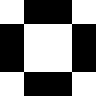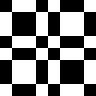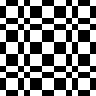Because of the mesmerizing non-regularity, for large N, your eyes may have a hard time staying focused.

64. Repetition words. Write a program Repetition.java to read in a list of dictionary words and print out all words for which each letter appears exactly twice, e.g., intestines, antiperspirantes, appeases, arraigning, hotshots, arraigning, teammate, and so forth.
65. Text twist. Write a program TextTwist.java that reads in a word from the command line and a dictionary of words from standard input, and prints all words of at least four letters that can be formed by rearranging a subset of the letters in the input word. This forms the core of the game Text Twist. Hint: create a profile of the input word by counting the number of times each of the 26 letters appears. Then, for each dictionary word, create a similar profile and check if each letter appears at least as many times in the input word as in the dictionary word.
66. Word frequencies. Write a program (or several programs and use piping) that reads in a text file and prints a list of the words in decreasing order of frequency. Consider breaking it up into 5 pieces and use piping: read in text and print the words one per line in lowercase, sort to bring identical words together, remove duplicates and print count, sort by count.
67. VIN numbers. A VIN number is a 17-character string that uniquely identifies a motor vehicle. It also encodes the manufacturer and attributes of the vehicle. To guard against accidentally entering an incorrect VIN number, the VIN number incorporates a check digit (the 9th character). Each letter and number is assigned a value between 0 and 9. The check digit is chosen so to be the weighted sum of the values mod 11, using the symbol X if the remainder is 10.
 A B C D E F G H I J K L M N O P Q R S T U V W X Y Z 1 2 3 4 5 6 7 8 - 1 2 3 4 5 - 7 - 9 2 3 4 5 6 7 8 9 1st 2nd 3rd 4th 5th 6th 7th 8th 9th 10 11 12 13 14 15 16 17 8 7 6 5 4 3 2 10 0 9 8 7 6 5 4 3 2 

For example the check digit of the partial VIN number 1FA-CP45E-?-LF192944 is X because the weighted sum is 373 and 373 mod 11 is 10.

  1 F A C P 4 5 E X L F 1 9 2 9 4 4 1 6 1 3 7 4 5 5 - 3 6 1 9 2 9 4 4 8 7 6 5 4 3 2 10 - 9 8 7 6 5 4 3 2 ------------------------------------------------------------------ 8 42 6 15 28 12 10 50 - 27 48 7 54 10 36 12 8 

Write a program VIN.java that takes a command line string and determines whether or not it is a valid VIN number. Allow the input to be entered with upper or lower case, and allow dashes to be inserted. Do thorough error checking, e.g., that the string is the right length, that no illegal characters are used (I, O, Q), etc.

68. Music CDs. Screen-scrape MusicBrainz to identify information about music CDs.
69. Pig Latin. Pig Latin is a fun secret language for young children. To convert a word to Pig Latin:
• If it begins with a vowel, append "hay" to the end. At the beginning of a word, treat y as a vowel unless it is followed by a vowel.
• If it begins with a sequence of consonants, move the consonants to the end, then append "ay". Treat a u following a q as a consonant.

For example, "input" becomes "input-hay", "standard" becomes "andard-stay", "quit" becomes "it-quay". Write a program PigLatinCoder.java that reads in a sequence of words from standard input and prints them to standard output in Pig Latin. Write a program PigLatinDecoder.java that reads in a sequence of words encoded in Pig Latin from standard input and prints the original words out in.

70. Rotating drum problem. Applications to pseudo-random number generators, computational biology, coding theory. Consider a rotating drum (draw picture of circle divided into 16 segments, each of one of two types - 0 and 1). We want that any sequence of 4 consecutive segments to uniquely identify the quadrant of the drum. That is, every 4 consecutive segments should represent one of the 16 binary numbers from 0000 to 1111. Is this possible? A de Bruijn sequence of order n is a shortest (circular) string such that every sequence of n bits appears as a substring at least once. For example, 0000111101100101 is a de Bruijn sequence of order 4, and all 2^4 possible 4-bit sequence (0000, 0001, ..., 1111) occur exactly once. Write a program DeBruijn.java that reads in a command line parameter n and prints an order n de Bruijn sequence. Algorithm: start with n 0's. Append a 1 if the n-tuple that would be formed has not already appeared in the sequence; append a 0 otherwise. Hint: use the methods String.indexOf and String.substring.
71. Ehrenfecucht-Mycielski sequence. The Ehrenfecucht-Mycielski sequence in a binary sequence that starts with "010". Given the first n bits b0, b1, ..., bn-1, bn is determined by finding the longest suffix bj, bj+1, ..., bn-1 that occurs previously in the sequence (if it occurs multiple times, take the last such occurrence). Then, bn is the opposite of the bit that followed the match. 0100110101110001000011110110010100100111010001100000101101111100. Hint: Use the substring() and lastIndexOf() methods.

#### Web Exercises (Image Processing)

1. Painter's and printer's color triangles. Create the following two images. The primary hues of the painter's triangle are red, green, and blue; the primary hues of the printer's triangle are magenta, cyan, and yellow.2. Two-stroke apparent motion. Create the optical illusion of two-stroke apparent motion or four-stroke
3. De Valois' checkerboard. Create the optical illusion of De Valois' checkerboard or one of the other optical illusions from the Shapiro Perception Lab.
4. Color spectrum. Write a program Spectrum.java that draws all 2^24 possible colors, by drawing for each red value a 256-by-256 array of color chips (one for each green and blue value).
5. Vertical flip. Write a program FlipY.java that reads in an image and flips it vertically.
6. Picture dimensions. Write a program Dimension.java that take the name of an image file as a command line input and prints its dimension (width-by-height).
7. Anti-aliasing. Anti-aliasing is a method of removing artifacts from representing a smooth curve with a discrete number of pixels. A very crude way of doing this (which also blurs the image) is to convert an N-by-N grid of pixels into an (N-1)-by-(N-1) by making each pixel be the average of four cells in the original image as below. Write a program AntiAlias that reads in an integer N, then an N-by-N array of integers, and prints the anti-aliased version. Reference.

8. Thresholding. Write a program Threshold.java that reads in a grayscale version of a black-and-white picture, creates and plots a histogram of 256 grayscale intensities, and determines the threshold value for which pixels are black, and which are white.
9. Mirror image. Read in a W-by-H picture and produce a 2W-by-H picture which concatenates the original W-by-H picture with the mirror image of the W-by-H picture. Repeat by mirror around the y-axis. Or create a W-by-H picture, but mirror around the center, deleting half the picture.
10. Linear filters. A box filter or mean filter replaces the color of pixel (x, y) by the average of its 9 neighboring pixels (including itself). The matrix [1 1 1; 1 1 1; 1 1 1] / 9 is called the convolution kernel. The kernel is the set of pixels to be averaged together. Program MeanFilter.java implements a mean filter using the Picture data type.
11. Blur filter. Use low-pass 3-by-3 uniform filter [1/13 1/13 1/13; 1/13 5/13 1/13; 1/13, 1/13, 1/13].
12. Emboss filter. Use prewitt masks [-1 0 1; -1 1 1; -1 0 1] (east) or [1 0 -1; 2 0 -2; 1 0 -1], [-1 -1 0; -1 1 1; 0 1 1] (south-east),
13. Sharpen filter. Psychophysical experiments suggest that a photograph with crisper edges is more aesthetically pleasing than exact photographic reproduction. Use a high-pass 3-by-3 filter. Light pixels near dark pixels are made lighter; dark pixels near light pixels are made darker. Laplace kernel. Attempts to capture region where second derivative is zero. [-1 -1 -1; -1 8 -1; -1 -1 -1]
14. Oil painting filter. Set pixel (i, j) to the color of the most frequent value among pixels with Manhattan distance W of (i, j) in the original image.
15. Luminance and chrominance. Decompose a picture using the YIQ color space: Y (luma) = 0.299 r + 0.587 g + 0.114 b, I (in-phase) = 0.596 r - 0.274 g - 0.322 b, and Q (quadrature) = 0.211 r - 0.523 g + 0.312 b. Plot all 3 images. The YIQ color space is used by NTSC color TV system.
16. Brighten. Write a program Brighter.java that takes a command line argument which is the name of a JPG or PNG file, displays it in a window, and display a second version which is a brighter copy. Use the Color method brighter(), which return a brighter version of the invoking color.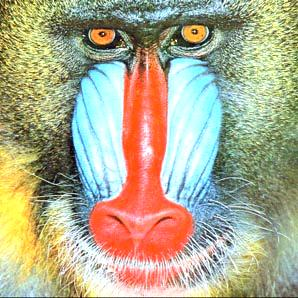17. Edge detection. Goal: form mathematical model of some feature of the image. To accomplish this, we want to detect edges or lines. An edge is a area of a picture with a strong contrast in intensity from one pixel to the next. Edge detection is a fundamental problem in image processing and computer vision. The Sobel method is a popular edge detection technique. We assume that the image is grayscale. (If not, we can convert by taking the average of the red, green, and blue intensities.) For each pixel (i, j) we calculate the edge strength by computing two 3-by-3 convolution masks. This involves taking the grayscale values of the nine pixels in the 3-by-3 neighborhood centered on (i, j), multiplying them by the corresponding weight in the 3-by-3 mask, and summing up the products.
 -1 0 +1 +1 +2 +1 -2 0 +2 0 0 0 -1 0 +1 -1 -2 -1 
This produces two values Gx and Gy. In the output picture, we color the pixel (i, j) according to the grayscale value 255 - Sqrt(Gx*Gx + Gy*Gy). There are various ways to handle the boundary. For simplicity, we ignore this special case and color the boundary pixels black. Program EdgeDetector.java takes the name of an image as a command line input and applies the Sobel edge detection algorithm to that image.